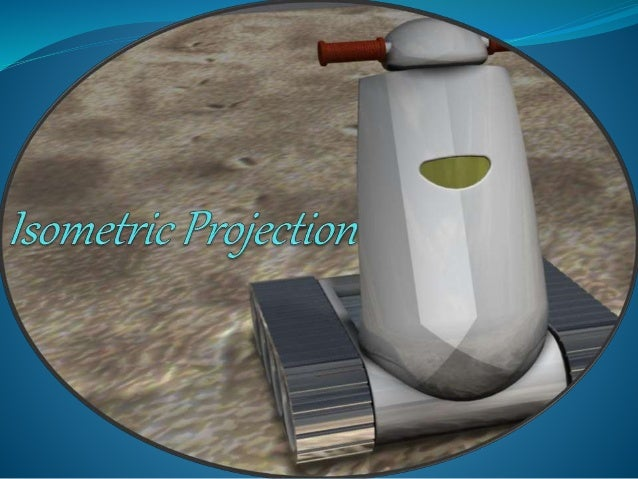Successfully reported this slideshow.Upcoming SlideShare
×

# ISOMETRIC PPT

17,489 views

Published on

i have created my own ppt on the topic name Isometric Projection.its a topic which is in engg. graphics book.

Published in: Engineering
• Full Name
Comment goes here.

Are you sure you want to Yes No• Thank you! It helps a lot\

Are you sure you want to  Yes  No

### ISOMETRIC PPT

1. 1. Isometric projections A cube shown with this type of projection pivots 45º to show two faces on the projection plane instead of a single face. It is then tilted frontward or backward, allowing three faces to be observed.
2. 2. Important Terms
3. 3. Content  Overview.  Pictorial projection  Parallel projection  Axonometric projection  Isometric projection.  Axes and selection  Isometric lines and planes  Isometric scale  Isometric projection & Isometric drawing  Producing Isometric sketches & drawing.  Isometric lines & non-isometric lines  Circles and arcs  Irregular curves  Oblique projection drawing.
4. 4. Pictorial projection  Pictorial projection:  Not intended to give exact or true view.  Not intended to transmit dimensions, although sometimes dimension is useful.  Useful when the information and instructions to be given to non-technical and untrained people.  Hidden lines are not shown in isometric
5. 5. Axonometric projection  axon = axis; metric = measure, in Greek  Axonometric projection is a parallel projection technique to create a pictorial drawing of an object by rotating the object on an axis relative to a projection or picture plane
6. 6.  Axonometric projection  Trimetric.  Dimetric.  Isometric. Axonometric projection
7. 7. Isometric projection  Isometric projection is a true representation of the isometric view of an object.  Isometric view is created by rotating the object 45 degree about vertical axis, and tilting it forward 35 deg 16’.
8. 8. Isometric projection: axes  The 3 axis meet at A,B form equal angles of 120 deg and they are called Isometric Axes  OA is vertical, OB is inclined at 30deg to the right, OC is inclined at 30deg to the left  Any lines parallel to these – Isometric Line  Any planes parallel – Isometric Planes
9. 9. Isometric projection: scale  The tilt causes the edges & planes to become foreshortened.  The projected length is approximately 80% of the true length.
10. 10. Draw the isometric projection of a rectangle of 100mm and 70mm sides if its plane is (a) Vertical and (b) Horizontal. To draw the isometric projection of a square plane Construction Case: Vertical plane  Draw a line at 30° to the horizontal and mark the isometric length on it.  Draw verticals at the ends of the line and mark the isometric length on these parallel lines.  Join the ends by a straight line which is also inclined at 30° to the horizontal.  There are two possible positions for the plane. Isometric Projection of Planes
11. 11. Case: Horizontal plane  Draw two lines at 30° to the horizontal and mark the isometric length along the line.  Complete the figure by drawing 30° inclined lines at the ends till the lines intersect. Note  (i) The shape of the isometric projection or drawing of a square is a Rhombus.  (ii) While dimensioning an isometric projection or isometric drawing true dimensional values only must be used.
12. 12. *The figure below shows how the cases can be executed.
13. 13. Isometric angles & non-isometric lines  Example of producing non- isometric lines.  The position of point Z is obtained in the isometric view, by transferring the distance of X and Y.
14. 14. Iso-circles and arcs: draw  Drawing isometric circles and arcs using four-centre method.
15. 15. Drawing iso-circles • To draw an iso-circle, on left plane, Diameter 20mm (a) Draw centre lines, vertical & 30deg to left. (b) Draw (construction line) 20mm “square box”. The centre lines should divide each side by half. (c) Draw straight lines; 1-2 & 1-3 and 2-5 & 2-6. (d) Point 7 is the intersection between line 1-2 & 2-5, and similarly point 8, 1-3 & 2-6 on the other side. (e) Set your compass to the distance 7-2, draw an arc with centre at point 7, from point 2 to point 5. Do the same on the other side. (f) Set your compass to the distance 1-2, draw an arc with centre (1), from (2) to (3). (a) 12 3 4 5 6 7 8 2 5 4 5 6 (b) (c) (d) (e) (f)
16. 16. Producing Isometric Projection  Read multi-view drawing given.  Observe scale, dimension, projection. angle  Determine front, side & top view.  Try to visualize how the object looks like.  Start with sketching, do not draw straight away.  If not sure, start with sketching an isometric box, enclosing the whole object.  You can label points,
17. 17. 6.3 Sketching isometric cylinder  Start by drawing the bounding box.  The front end of the cylinder is sketched using the previous technique.  The far end of the cylinder is a partial iso-circle. Sketch until meeting the tangent with the two straight lines.
18. 18. Isometric Projection ORTHOGRAPHIC MULTI-VIEW PROJECTION ISOMETRIC PROJECTION «Construction box» How to draw an object containing rounded parts
19. 19. THANK YOU Department : Mechanical M-1 (B-Batch). Created by : Parth P. Prajapati.